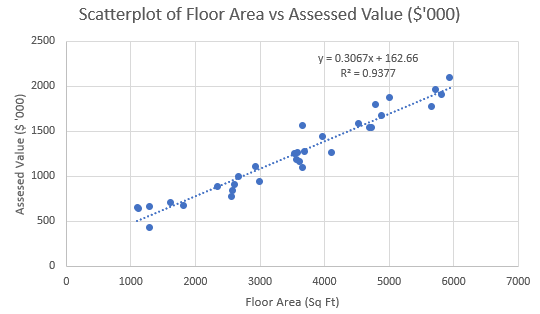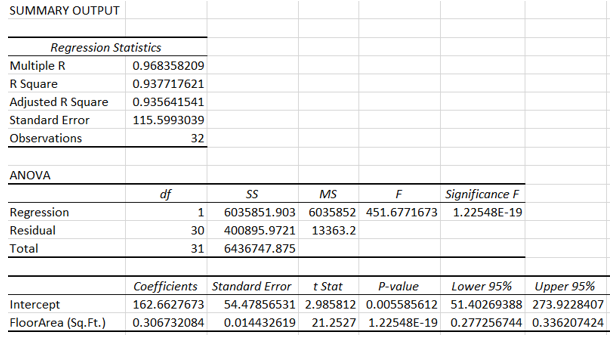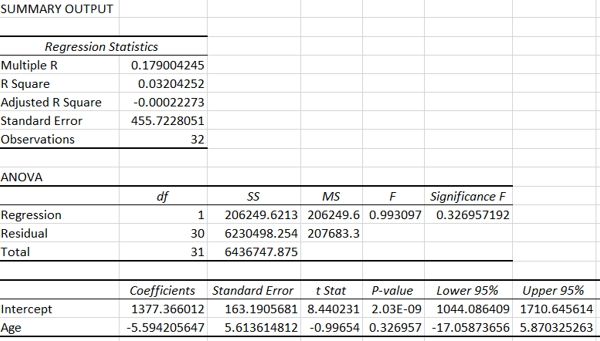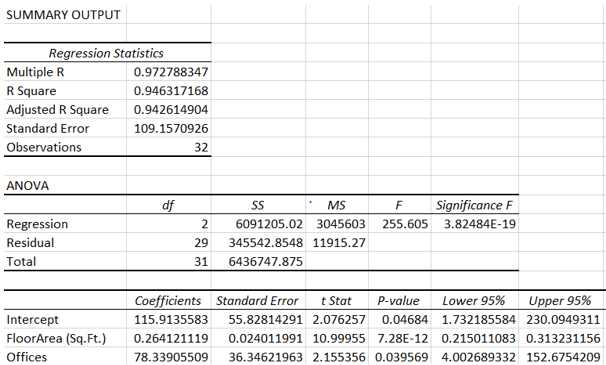# Fall 2022 Mid-Term Exam Solution on Regression Analysis- SPSS from the University of Cambridge

The following is a coding question presented during the fall 2022 mid-term exam on regression analysis – SPSS examined at the University of Cambridge. Attached to it are possible solutions that explain the theory clearly. If you need help with your statistics exam, don't hesitate to pay us to take your regression analysis exam. Get in touch with us now.

## Exam Question: explain the linear and multiple regression analysis

Exam Solution:
This paper will analyze the relationship between the tax-assessed value of a property and floor area, the number of offices in the building, the number of customer entrances and the age of the building. Simple linear regression and multiple regression models were created to model the relationship between the variables. Other than this, feel free to hire our experienced statistics exam doers to complete your regression analysis exam.
The following scatterplot shows the plot of floor area vs assessed value (in $' 000) for the properties in the dataset. Figure 1: Scatterplot of floor area and assessed value.The scatterplot shows a linear relationship between floor area and the property's assessed value. The R-squared value of the regression model is 0.9377, which suggests a strong linear relationship between the two variables. The following figure shows the linear regression output between floor area and property assessment value. Figure 2: Regression output of Assessment value and floor area.The F-statistic is equal to 451.68, and the p-value is 1.225*10-19 < 0.05. Thus, the null hypothesis is rejected, and the linear regression is statistically significant. Thus, floor The following scatter plot shows the relationship between the age of a building and the assessed value. Figure 3: Scatterplot of the age of the building and assessed value.The scatterplot provides very little evidence to support the hypothesis of a linear relationship between the two variables. The R2 value of 0.032 indicates little support for a linear relationship between the two variables. Figure 4: Regression output of age of building and floor area.The p-value of the Age variable is 0.327 > 0.05. Thus, one fails to reject the null hypothesis, and it concludes that age is not a statistically significant predictor of assessed value. The following figure shows the output of the multiple regression analysis Figure 5: Output of multiple regression model.The overall r2 value of the model is 0.953, while the adjusted r2 value is 0.946. At a significance level of 0.05, floor area and the number of offices are statistically significant, as their p-values are less than 0.05. The number of customer entrances and the age of the building can be eliminated. The following figure shows the output of a multiple regression model, with only floor area and the number of offices as predictor variables. Figure 6: Multiple regression outputsThe final model is Assessed Value = 115.9 + 0.26*Floor Area + 78.34*Offices The assessed value of a medical office building with a floor area of 3500 Sq.Ft. and 2 offices is$2,215,423.302. The predicted assessed value is not consistent with the database as the highest assessed value is \$2.094 million, but the predicted value lies outside the range of the assessed values.